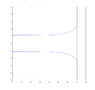# Parabolic Pressure Distribution in a Jet

person123
Assume the jet is straight but the radius of the jet varies over it's length (like a jet of water falling which narrows due to gravitational acceleration). Also ignore viscosity. A pressure gradient would be required to accelerate the fluid radially. Because during an expansion transformation, the distance a point moves (and so its acceleration) is proportional to its distance from the origin, the radial acceleration of fluid parcels would be proportional to their distance from the middle of the jet. The radial acceleration is proportional to the radial pressure gradient. This would mean the pressure gradient would be proportional to the distance from the middle, which would mean the pressure distribution is parabolic (the integral of a linear function is quadratic).

This was my reasoning; however I don't know my assumptions are reasonable and if the conclusion is accurate, so I would be interested in people's input. Thanks!

Mentor
The neglect of viscosity is the fallacy in your reasoning. The radial component of the stress is equal to atmospheric pressure throughout the jet cross section. It is viscous stress (from the 3D version of Newton's law of viscosity) that is responsible for the jet contraction.

person123
Thank you for the response.
If the fluid was truly inviscid, what would occur? Would this just not be a meaningful scenario, or would the flow sort of detach (I'm not sure what this would physically entail), speeding up while not converging?

Mentor
Continuity requires that if the flow speeds up, it must converge. Viscosity makes this happen.

person123
Would it be possible to have an inviscid fluid undergoing these conditions?

Mentor
Would it be possible to have an inviscid fluid undergoing these conditions?
If the surface tension were high enough.

•person123
person123
Thank you for the responses that makes sense. In the case of flow converging as it falls, I imagine this would be seen clearly when the flow is laminar and viscous forces and surface tension dominates.

I hope I'm not going into to many "what ifs," but would the pressure forces dominate for highly Reynold's number flow, such as a fast jet impacting a surface (with the jet normal to the surface)?

I wrote a simple code in MatLab to model the flow of a jet impacting a surface by following a particle on the boundary where I would assume the speed is constant. I had the code find the radial speed using what I would imagine the radial pressure gradient might be, find the average jet speed through continuity, find the new average pressure through Bernoulli's equation, and use that to find the pressure gradient assuming the average pressure is proportional to the pressure gradient (and of course repeat in a loop). I attached the result.

#### Attachments

•JetImpact.png
2.5 KB · Views: 77
Mentor
Thank you for the responses that makes sense. In the case of flow converging as it falls, I imagine this would be seen clearly when the flow is laminar and viscous forces and surface tension dominates.

I hope I'm not going into to many "what ifs," but would the pressure forces dominate for highly Reynold's number flow, such as a fast jet impacting a surface (with the jet normal to the surface)?

I wrote a simple code in MatLab to model the flow of a jet impacting a surface by following a particle on the boundary where I would assume the speed is constant. I had the code find the radial speed using what I would imagine the radial pressure gradient might be, find the average jet speed through continuity, find the new average pressure through Bernoulli's equation, and use that to find the pressure gradient assuming the average pressure is proportional to the pressure gradient (and of course repeat in a loop). I attached the result.
This sounds very ad hoc. I would very much prefer seeing the proper fluid dynamic partial differential equations for continuity, momentum balance (Euler or Navier Stokes), and appropriate expression of the boundary condition at the free surface, and then any assumptions and their application to simplify the equations (appropriately justified). Any approach following individual particles, in my judgment, is inappropriate for a complicated system like this.

person123
Let's say I assume steady, inviscid, incompressible flow with no body forces. I could also assume radial symmetry, which I imagine would allow things to be simplified. This might allow me to express the equations in cylindrical coordinates, making it a 2d problem (eliminating theta).

The equations would then reduce to: $$\nabla \cdot u=0$$ $$\frac{\nabla p}{\rho}+u\cdot \nabla u=0$$
I'm guessing I would have three boundary conditions, one for the free surface, one for the surface, and one for the cross section of the start of the jet where the velocity is assumed to be uniform. This is my guess at the boundary conditions, but I'm not sure if they're correct.

Free surface where ##u_n## is normal to the free surface (I'm not sure having both is redundant): $$p=0$$ $$u_n=0$$

Plate where ##u_z## is normal to the plate (so in direction of jet):$$u_z=0$$

Jet cross-section: $$u_z=v_{jet}$$ $$u_r=0$$

I'm guessing you could then use the potential function to get a system of differential equations with a single variable and solve it with those boundary conditions. I imagine this would be very difficult, but it would be interesting to see what it gives me and how it compares to my other approach.

Last edited:
•Chestermiller